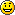# Rebuilding TCP streams with Ruby part 2: fuzzysort

March 19, 2008

This is part 2 of a series on rebuilding TCP streams using Ruby, for more information, visit the previous post:

In my previous post, I talked about using fuzzy sequence/acknowledge numbers to split a network capture file into streams. Using fuzzymatch was pretty successful for cutting streams out, but the streams themselves were not ordered. This version of the Ruby StreamBuilder library orders the streams by using increasing seq/ack numbers, or as I like to call it “fuzzysort”. In order to do this, fuzzysort first splits the stream into a “source” stream and a “destination” stream. After spliting the streams, the streams are ordered in ascending acknowledgement order, where if there are duplicate acks, the ascending sequence numbers are used. The streams are then printed in ordered fashion (since this is just proof of concept)

It’s interesting to note that because of implementing the ordered streams in a hash using the seq/ack as the key, the list does not handle duplicate packets. I added some logic so that a large data packet is not replaced by a simple ack packet with the same numbers, so the streams should still have the correct data after being ordered.

Here’s an example of running fuzzymatch-sort.rb on a randomized single-stream pcap file:

```shell> ./fuzzymatch-sort.rb ../pcaps/pLargeRand.pcap     [....S.] 128.222.228.89 -> 128.222.228.77    seq=638858703 ack=0 len=78 Starting a new stream...     [.A..S.] 128.222.228.77 -> 128.222.228.89    seq=2849933258 ack=638858704 len=74 ack num: 638858704 close enough to 638858703 to add. Had to check 1 streams and 2 seq/ack nums     [.A....] 128.222.228.89 -> 128.222.228.77    seq=638862706 ack=2849933260 len=66 ack num: 2849933260 close enough to 2849933258 to add. Had to check 1 streams and 2 seq/ack nums     [.AP...] 128.222.228.89 -> 128.222.228.77    seq=638861176 ack=2849933259 len=666 ack num: 2849933259 close enough to 2849933260 to add. Had to check 1 streams and 1 seq/ack nums     [.A....] 128.222.228.89 -> 128.222.228.77    seq=638858704 ack=2849933259 len=66 ack num: 2849933259 close enough to 2849933259 to add. Had to check 1 streams and 1 seq/ack nums     [.A....] 128.222.228.77 -> 128.222.228.89    seq=2849933259 ack=638861776 len=66 seq num: 2849933259 close enough to 2849933259 to add. Had to check 1 streams and 1 seq/ack nums     [.A....] 128.222.228.89 -> 128.222.228.77    seq=638859728 ack=2849933259 len=1514 ack num: 2849933259 close enough to 2849933259 to add. Had to check 1 streams and 2 seq/ack nums     [.A....] 128.222.228.77 -> 128.222.228.89    seq=2849933259 ack=638862706 len=66 seq num: 2849933259 close enough to 2849933259 to add. Had to check 1 streams and 3 seq/ack nums     [.AP...] 128.222.228.89 -> 128.222.228.77    seq=638858704 ack=2849933259 len=1090 ack num: 2849933259 close enough to 2849933259 to add. Had to check 1 streams and 3 seq/ack nums     [.A....] 128.222.228.77 -> 128.222.228.89    seq=2849933259 ack=638861176 len=66 seq num: 2849933259 close enough to 2849933259 to add. Had to check 1 streams and 3 seq/ack nums     [.A...F] 128.222.228.77 -> 128.222.228.89    seq=2849933259 ack=638862706 len=66 seq num: 2849933259 close enough to 2849933259 to add. Had to check 1 streams and 3 seq/ack nums Ended up with 1 stream(s).```

```==> Stream 1 contains 11 packet(s) --> Sorting stream 1...     [....S.] 128.222.228.89 -> 128.222.228.77    seq=638858703 ack=0 len=78     [.A..S.] 128.222.228.77 -> 128.222.228.89    seq=2849933258 ack=638858704 len=74     [.A....] 128.222.228.89 -> 128.222.228.77    seq=638862706 ack=2849933260 len=66     [.AP...] 128.222.228.89 -> 128.222.228.77    seq=638861176 ack=2849933259 len=666     [.A....] 128.222.228.89 -> 128.222.228.77    seq=638858704 ack=2849933259 len=66     [.A....] 128.222.228.77 -> 128.222.228.89    seq=2849933259 ack=638861776 len=66     [.A....] 128.222.228.89 -> 128.222.228.77    seq=638859728 ack=2849933259 len=1514     [.A....] 128.222.228.77 -> 128.222.228.89    seq=2849933259 ack=638862706 len=66     [.AP...] 128.222.228.89 -> 128.222.228.77    seq=638858704 ack=2849933259 len=1090    [.A....] 128.222.228.77 -> 128.222.228.89    seq=2849933259 ack=638861176 len=66    [.A...F] 128.222.228.77 -> 128.222.228.89    seq=2849933259 ack=638862706 len=66```

`==== Unsorted Streams ====`

```Source ------- [....S.] 128.222.228.89 -> 128.222.228.77    seq=638858703 ack=0 len=78 [.A....] 128.222.228.89 -> 128.222.228.77    seq=638862706 ack=2849933260 len=66 [.AP...] 128.222.228.89 -> 128.222.228.77    seq=638861176 ack=2849933259 len=666 [.A....] 128.222.228.89 -> 128.222.228.77    seq=638858704 ack=2849933259 len=66 [.A....] 128.222.228.89 -> 128.222.228.77    seq=638859728 ack=2849933259 len=1514 [.AP...] 128.222.228.89 -> 128.222.228.77    seq=638858704 ack=2849933259 len=1090 Dest ------- [.A..S.] 128.222.228.77 -> 128.222.228.89    seq=2849933258 ack=638858704 len=74 [.A....] 128.222.228.77 -> 128.222.228.89    seq=2849933259 ack=638861776 len=66 [.A....] 128.222.228.77 -> 128.222.228.89    seq=2849933259 ack=638862706 len=66 [.A....] 128.222.228.77 -> 128.222.228.89    seq=2849933259 ack=638861176 len=66 [.A...F] 128.222.228.77 -> 128.222.228.89    seq=2849933259 ack=638862706 len=66```

`==== Sorted Streams ====`

```Source ------- [....S.] 128.222.228.89 -> 128.222.228.77    seq=638858703 ack=0 len=78 [.AP...] 128.222.228.89 -> 128.222.228.77    seq=638858704 ack=2849933259 len=1090 [.A....] 128.222.228.89 -> 128.222.228.77    seq=638859728 ack=2849933259 len=1514 [.AP...] 128.222.228.89 -> 128.222.228.77    seq=638861176 ack=2849933259 len=666 [.A....] 128.222.228.89 -> 128.222.228.77    seq=638862706 ack=2849933260 len=66 Dest ------- [.A..S.] 128.222.228.77 -> 128.222.228.89    seq=2849933258 ack=638858704 len=74 [.A....] 128.222.228.77 -> 128.222.228.89    seq=2849933259 ack=638861176 len=66 [.A....] 128.222.228.77 -> 128.222.228.89    seq=2849933259 ack=638861776 len=66 [.A...F] 128.222.228.77 -> 128.222.228.89    seq=2849933259 ack=638862706 len=66```

Another addition in this version is that the fuzzymatch algorithm that is used to generate streams has been optimized (mostly because I was doing it stupidly last time) so that seq/ack searches don’t take nearly as long for large pcap files. You can download an example of fuzzysort output from a pcap file with 5 streams here.

While this is a step up from the unsorted fuzzymatch from the last post, this method still has its downfalls, not being able to store duplicate seq/ack packets could definitely cause problems. The ability to generate a sorted stream without having to split the stream into source and destination streams would also be extremely useful (so you could see a full transmission stream from both sides).

The next evolution of the library would be to actually keep a state table and follow TCP streams using the seq/ack numbers (which I attempted for this version, but it was extremely complex, so I scraped it and did fuzzysort). Hopefully I’ll be able to implement it without any problems. I might take the easy route and go for using TCP TSVal to attempt to order streams.

Comments? Criticism? Leave a comment and let me know### 1 Comment to "Rebuilding TCP streams with Ruby part 2: fuzzysort"

1. […]  Rebuilding TCP streams with Ruby part 2: fuzzysort […]